# pcbStack

Single feed or multi-feed PCB antenna

## Description

The `pcbStack` object is a single feed or multi-feed printed circuit board (PCB) antenna. Use the `pcbStack` object:

• To create single-layer, multilayer metal, or metal-dielectric substrate antennas.

• To create an arbitrary number of feeds and vias in an antenna.

• To create a PCB antenna from antenna and array catalog elements.

Note

To generate a Gerber file, a substrate layer is required. Use the `Substrate` property to create this layer in the PCB antenna. For more information, see Convert Circular Microstrip Patch into PCB Antenna

## Creation

### Syntax

``pcbant = pcbStack``
``pcbant = pcbStack(Name=Value)``
``pcbant = pcbStack(ant)``

### Description

``` `pcbant = pcbStack` creates an air-filled single-feed PCB with two metal layers.```

example

``` `pcbant = pcbStack(Name=Value)` creates a PCB antenna, with additional Properties specified by one or more name-value arguments. `Name` is the property name and `Value` is the corresponding value. You can specify multiple name-value arguments in any order as `Name1`=`Value1`, `...`, `NameN`=`ValueN`. Properties not specified retain their default values.For example, `pcbStack(FeedDiameter=2.000e-04)` creates a PCB antenna with a feed diameter of 2.000e-04 meters.```

example

``` `pcbant = pcbStack(ant)` converts any 2-D or 2.5-D antenna from the antenna catalog into a PCB antenna for further modeling and analysis. You can also convert antenna array objects from the antenna array catalog to a PCB antenna. For a list of supported catalog elements, see Supported Catalog Elements.```

## Properties

expand all

Name of PCB antenna, specified a character vector.

Example: `"PCBPatch"`

Data Types: `string`

Revision details of PCB antenna design, specified as a character vector.

Example: `"2.0"`

Data Types: `string`

Shape of the PC board, specified as a shape object from: `antenna.Rectangle`, `antenna.Circle`, `antenna.Ellipse`, `antenna.Polygon`, or `antenna.Triangle`.

Example: `antenna.Polygon`

Thickness of the PC board, specified as a positive scalar. The value of this property is the sum of the thicknesses of all the dielectric layers that lie below the top metal layer. The object treats dielectric layers that are above the top metal layer as coating. Set this property before you set the `Layers` property. For more information on `BoardThickness`, see Board Thickness versus Dielectric Thickness in PCB.

Example: `0.02000`

Data Types: `double`

Metal and dielectric layers, specified a cell array of metal layer shapes and dielectric. You can specify one metal shape or one dielectric per layer starting with the top layer and proceeding down.

Data Types: `cell`

Feed locations for PCB antenna in Cartesian coordinates, specified as N -by-3 for a balanced feed or N-by-4 array for an unbalanced feed. You can place feed inside the board or at the edge of the board. The arrays translate to the following:

• N -by-3 – [x, y, Layer]

• N-by-4 – [x, y, SigLayer, GndLayer]

Antenna Toolbox™ uses Delta-Gap source feed model to excite the antenna structure. For more information, see Feed Model

Example: `[-0.0187 0 1 2]`

Data Types: `double`

Center pin diameter of feed connector, specified as a positive scalar in meters.

Example: `2.000e-04`

Data Types: `double`

Electrical short locations for antenna in Cartesian coordinates, specified as a real vector of size M-by-4 array. The arrays translate to the following:

• M-by-4 – [x, y, SigLayer, GndLayer]

Example: `[0 -0.025 1 2]`

Data Types: `double`

Electrical shorting pin diameter between metal layers, specified as a positive scalar in meters for a single pin or a positive vector in meters for multiple pins. Number of values specified in this property must match the number of pins.

Example: `1.0e-3`

Data Types: `double`

Magnitude voltage applied at the feeds, specified as a positive scalar in volts.

Example: `2`

Data Types: `double`

Model for approximating feed and via, specified as one of the following:

• `strip` – A rectangular strip approximation to the feed or via cylinder. This approximation is the simplest and results in a small mesh.

• `square` – A 4-sided polyhedron approximation to the feed or via cylinder.

• `hexagon` – A 6-sided polyhedron approximation to the feed or via cylinder.

• `octagon` – A 8-sided polyhedron approximation to the feed or via cylinder.

Example: `"octagon"`

Data Types: `string`

Excitation phase at each feed, specified as a real vector in degrees.

Example: `2`

Data Types: `double`

Type of the metal used as a conductor, specified as a metal material object. You can choose any metal from the `MetalCatalog` or specify a metal of your choice. For more information, see `metal`. For more information on metal conductor meshing, see Meshing.

Example: `metal("Copper")`

Lumped elements added to the antenna feed, specified as a lumped element object handle. For more information, see `lumpedElement`.

Example: `Load=lumpedelement`, where `lumpedelement` is the object handle for the load created using `lumpedElement` object.

Example: ```pcbant.Load = lumpedElement(Impedance=75)```

Tilt angle of the antenna in degrees, specified as a scalar or vector. For more information, see Rotate Antennas and Arrays.

Example: `90`

Example: `Tilt=[90 90]`,`TiltAxis=[0 1 0;0 1 1]` tilts the antenna at 90 degrees about the two axes defined by the vectors.

Data Types: `double`

Tilt axis of the antenna, specified as one of these values:

• Three-element vector of Cartesian coordinates in meters. In this case, each coordinate in the vector starts at the origin and lies along the specified points on the x-, y-, and z-axes.

• Two points in space, specified as a 2-by-3 matrix corresponding to two three-element vectors of Cartesian coordinates. In this case, the antenna rotates around the line joining the two points.

• `"x"`, `"y"`, or `"z"` to describe a rotation about the x-, y-, or z-axis, respectively.

Example: `[0 1 0]`

Example: `[0 0 0;0 1 0]`

Example: `"Z"`

Data Types: `double` | `string`

## Object Functions

 `show` Display antenna, array structures, shapes, or platform `array` Create array of PCB stack objects `axialRatio` Axial ratio of antenna `beamwidth` Beamwidth of antenna `charge` Charge distribution on antenna or array surface `current` Current distribution on antenna or array surface `efficiency` Radiation efficiency of antenna `EHfields` Electric and magnetic fields of antennas or embedded electric and magnetic fields of antenna element in arrays `impedance` Input impedance of antenna or scan impedance of array `info` Display information about antenna or array `layout` Display array or PCB stack layout `mesh` Mesh properties of metal, dielectric antenna, or array structure `meshconfig` Change meshing mode of antenna, array, custom antenna, custom array, or custom geometry `pattern` Plot radiation pattern and phase of antenna or array or embedded pattern of antenna element in array `patternAzimuth` Azimuth plane radiation pattern of antenna or array `patternElevation` Elevation plane radiation pattern of antenna or array `returnLoss` Return loss of antenna or scan return loss of array `sparameters` Calculate S-parameters for antennas and antenna arrays `vswr` Voltage standing wave ratio (VSWR) of antenna or array element `plot` Plot boundary of 2-D shape

## Examples

collapse all

Setup parameters.

```vp = physconst("lightspeed"); f = 850e6; lambda = vp./f;```

```L = 0.15; W = 1.5*L; stripL = L; gapx = 0.015; gapy = 0.01; r1 = antenna.Rectangle(Center=[0 0], Length=L, Width=W, Center=[lambda*0.35 0]); r2 = antenna.Rectangle(Center=[0 0], Length=L, Width=W, Center=[-lambda*0.35 0]); r3 = antenna.Rectangle(Length=0.5*lambda, Width=0.02*lambda, NumPoints=2); s = r1 + r2 + r3; figure show(s)```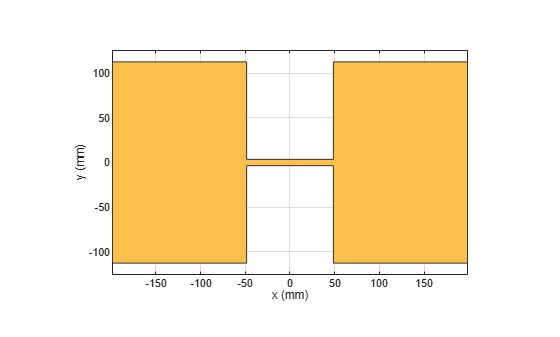Assign the radiator shape to pcbStack and make the changes to the board shape and feed diameter properties.

```boardShape = antenna.Rectangle(Length=0.6, Width=0.3); p = pcbStack; p.BoardShape = boardShape; p.Layers = {s}; p.FeedDiameter = 0.02*lambda/2; p.FeedLocations = [0 0 1]; figure show(p)```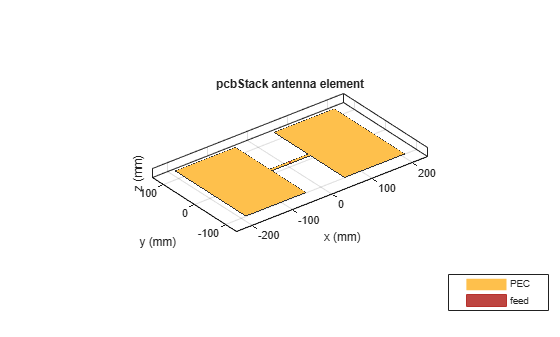Analyze the impedance of the antenna. Effect of the end-loading should result in the series resonance to be pushed lower in the band.

```figure impedance(p,linspace(200e6,1e9,51))```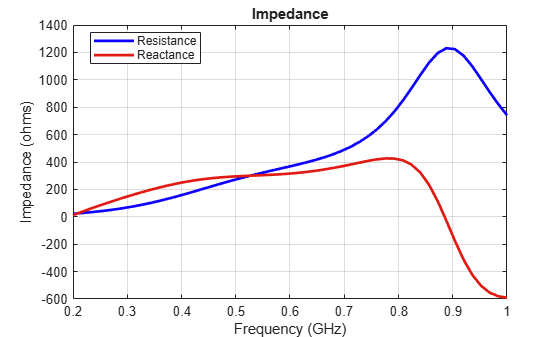Create a pcb stack antenna with 2 mm dielectric thickness at the radiator and air below it. Display the structure.

```p = pcbStack; d1 = dielectric("FR4"); d1.Thickness = 2e-3; d2 = dielectric("Air"); d2.Thickness = 8e-3; p.Layers = {p.Layers{1},d1,d2,p.Layers{2}}; p.FeedLocations(3:4) = [1 4]; show(p)```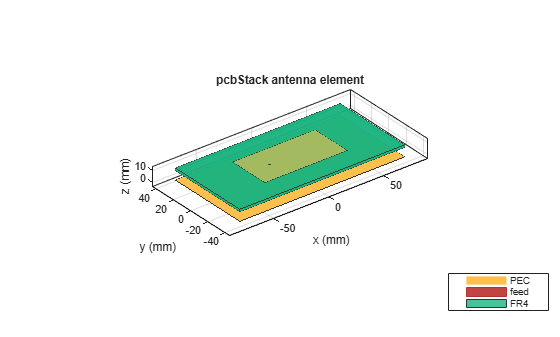Create a PCB stack antenna from reflector backed bowtie.

```b = design(bowtieRounded,1e9); b.Tilt = 90```
```b = bowtieRounded with properties: Length: 0.0959 FlareAngle: 90 Conductor: [1x1 metal] Tilt: 90 TiltAxis: [1 0 0] Load: [1x1 lumpedElement] ```
```b.TiltAxis = [0 1 0]; r = reflector(Exciter=b); p = pcbStack(r);```

Plot the directivity pattern of the antenna at 1 GHz.

`pattern(p,1e9);`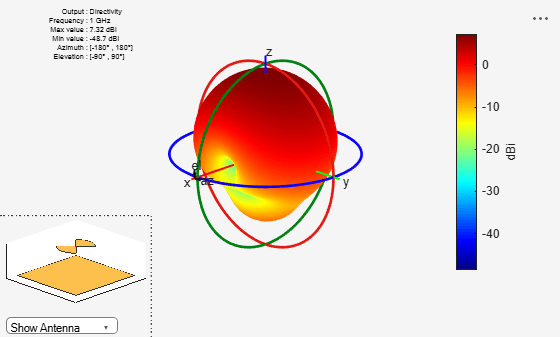Create a coplanar inverted F antenna.

```fco = invertedFcoplanar(Height=14e-3, GroundPlaneLength=100e-3,... GroundPlaneWidth=100e-3);```

Use this antenna to create a `pcbStack` object.

`p = pcbStack(fco);`

Create a circular microstrip patch.

```p = patchMicrostripCircular; d = dielectric; d.EpsilonR = 4.4; p.Radius = 0.0256; p.Height = 1.6e-3; p.Substrate = d; p.GroundPlaneLength = 3*0.0256; p.GroundPlaneWidth = 3*0.0256; p.FeedOffset = [0.0116 0];```

Create a PCB of this patch using `pcbStack`.

```pb = pcbStack(p); pb.FeedDiameter = 1.27e-3; pb.ViaLocations = [0 pb.FeedLocations(1)/1.1 1 3]; pb.ViaDiameter = pb.FeedDiameter; figure show(pb)```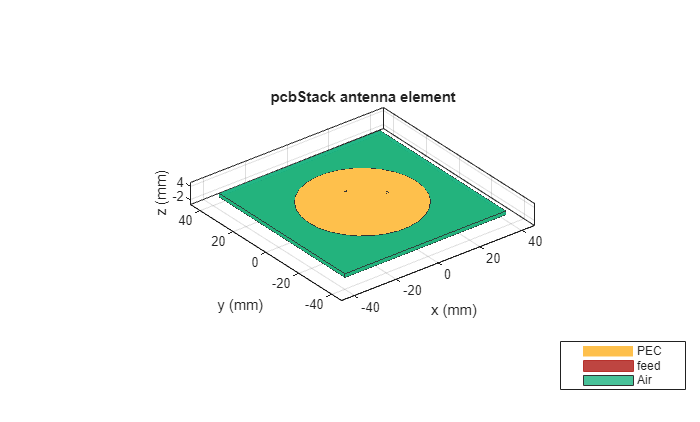Define the RF SMA connector and use it with the Mayhew writer service to generate Gerber files of the design.

```C = SMA_Jack_Cinch; O = PCBServices.MayhewWriter; O.DefaultViaDiam = pb.ViaDiameter; O.Filename = 'Microstrip circular patch-9a'; Am = PCBWriter(pb,O,C); gerberWrite(Am)```

Images using Mayhew Labs 3-D Viewer.Create a coplanar inverted-F antenna.

```fco = invertedFcoplanar(Height=14e-3, GroundPlaneLength=100e-3,... GroundPlaneWidth=100e-3); show(fco)```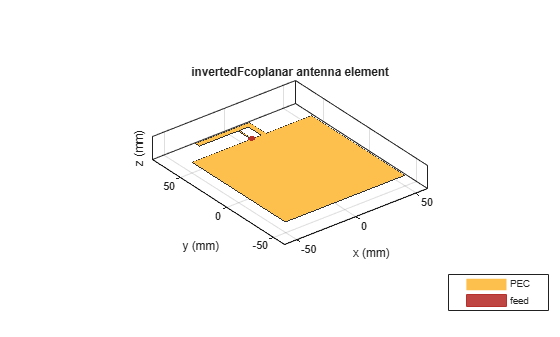Create a linear array with inverted-F antenna as its elements.

```la = linearArray; la.Element = fco; la.NumElements = 4; show(la)```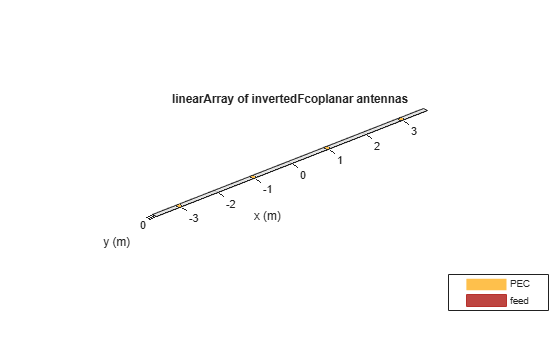Use this antenna array to create the PCB antenna.

```p = pcbStack(la); show(p)```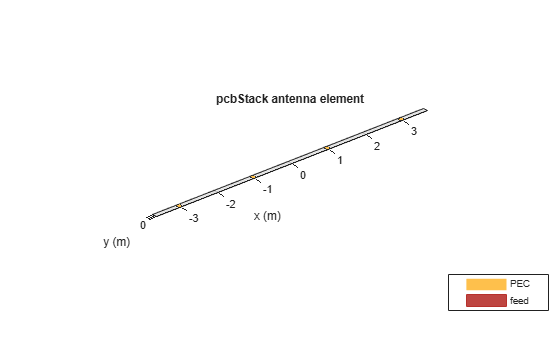Create a `dipole` antenna object and `linearArray` antenna array object. In the` linearArray` antenna object, leave the` Element` property set to its default value of dipole. Set the `ElementSpacing` property to 4."

```d1 = dipole; d2 = linearArray(ElementSpacing=4);```

To set the Z-coordinate of `pcbStack` antenna object to zero, rotate the dipole and linear dipole array around 90 degrees using the `Tilt `property. Then set the `TiltAxis` property to [ 0 -1 0 ] for dipole and linear dipole array antennas.

```d1.Tilt = 90; d2.Element.Tilt = 90; d1.TiltAxis = [0 -1 0]; d2.Element.TiltAxis = [0 -1 0];```

Create and view PCB stack antenna created using the `dipole` antenna object.

```p1 = pcbStack(d1); show(p1)```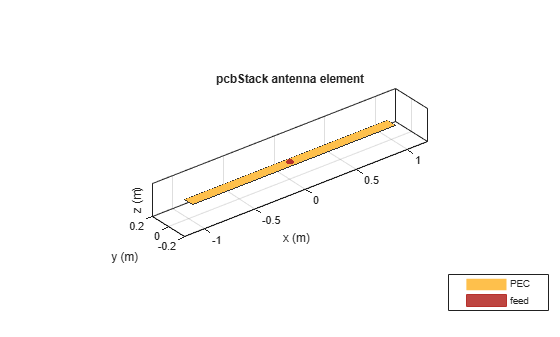Create and view PCB stack antenna using the `linearArray` antenna array object.

```p2 = pcbStack(d2); show(p2)```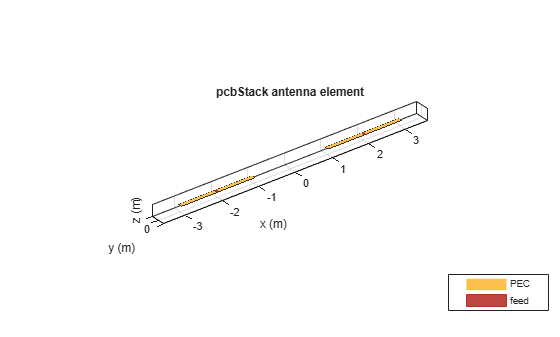Create a circular microstrip patch antenna.

```ant = design(patchMicrostripCircular,3e9); ant.Substrate = dielectric("FR4"); show(ant)```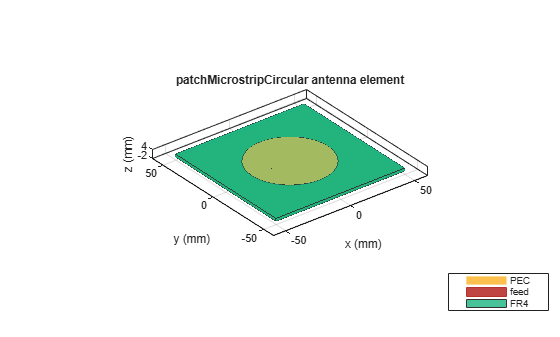```c = antenna.Circle; show(c)```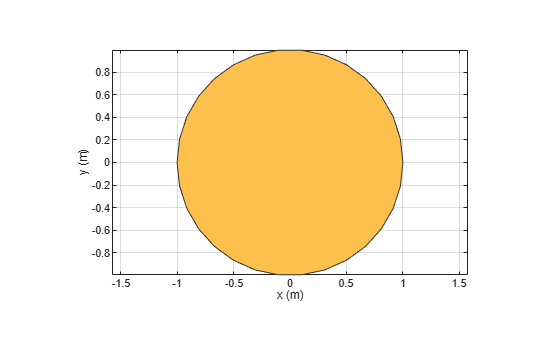```c.NumPoints = 6; c.Radius = 3*ant.Radius; figure show(c)```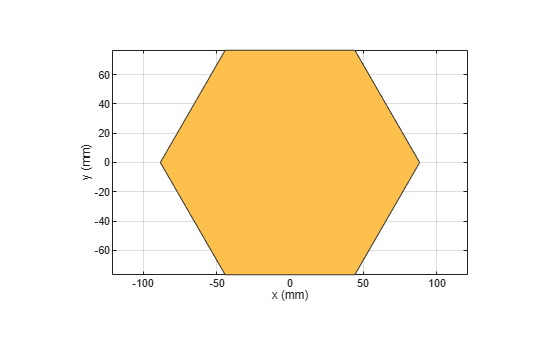Create the PCB stack using the vertices derived from the circle shape.

```v = getShapeVertices(c); cp = antenna.Polygon(Vertices=v); pb = pcbStack(ant); pb.Layers{3} = cp; pb.BoardShape = cp; show(pb) axis equal```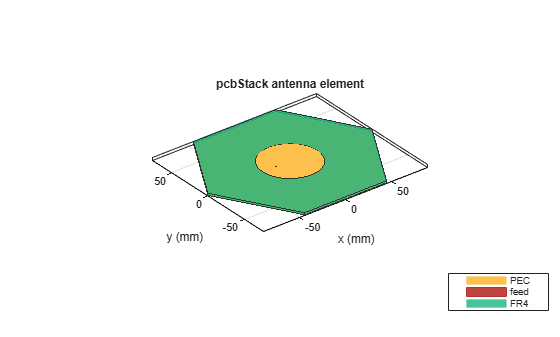## Supported Catalog Elements

The following antennas and arrays from the catalog can be converted into a PCB antenna by the `pcbStack` object:

Antennas

Note

Orient these antennas in the xy-plane if required, before conversion to `pcbStack`.

• Dipole Antennas: `dipole`, `bowtieTriangular`, `bowtieRounded`, and `dipoleBlade`.

• Fractal Antennas: `fractalCarpet`, `fractalGasket`, `fractalIsland`, `fractalKoch`, `fractalSnowflake`

• Loop Antennas: `loopCircular`, `loopRectangular`

• Monopole Antennas: `invertedFcoplanar`, `invertedLcoplanar`

• Patch Antennas: `patchMicrostrip`, `patchMicrostripCircular`, `patchMicrostripElliptical`, `patchMicrostripEnotch`, `patchMicrostripHnotch`, `patchMicrostripInsetfed`, `patchMicrostripTriangular`

• Reflector Antennas: `reflector` and `reflectorCircular` with a finite ground plane.

• Slot Antennas: `slot`, `vivaldi`, `vivaldiAntipodal`, `vivaldiOffsetCavity`

• Spiral Antennas: `spiralArchimedean`, `spiralEquiangular`, `spiralRectangular`

• Other Antennas: `lpda`, `customAntennaGeometry`, `customArrayGeometry`

Arrays

Note

The arrays must be homogeneous, with uniform elements.

• `linearArray`

• `circularArray`

• `rectangularArray`

 Balanis, C. A. Antenna Theory. Analysis and Design. 3rd Ed. Hoboken, NJ: John Wiley & Sons, 2005.

 Stutzman, W. L. and Gary A. Thiele. Antenna Theory and Design. 3rd Ed. River Street, NJ: John Wiley & Sons, 2013.